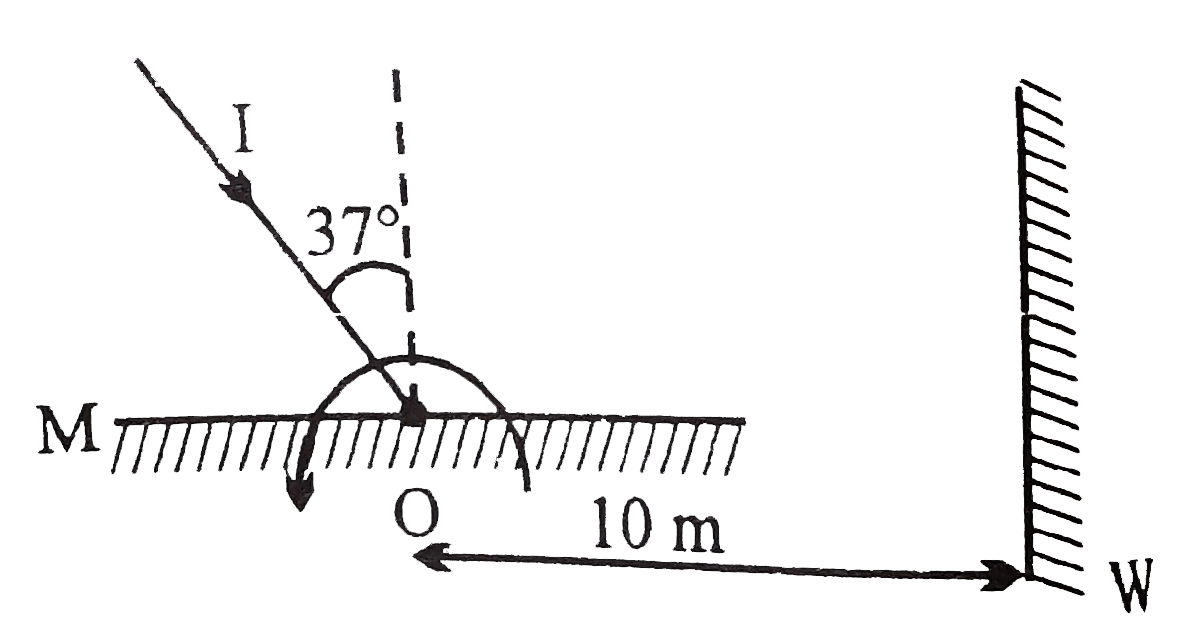# A light ray I is incident on a plane mirror M.The mirror is rotated in the direction as shown in the figure by an arrow at frequency (9)/(pi) "re

20 views
in Physics
A light ray I is incident on a plane mirror M.The mirror is rotated in the direction as shown in the figure by an arrow at frequency (9)/(pi) "rev"//"sec",The light reflected by the mirror is received on the wall W at a distance 10mfrom the axis of rotation .When the angle of incidence becomes 37^(@)`find the speed of the spot (a point) on the wall?A. 10 m/s
B. 1000 m/s
C. 500 m/s
D. 20 m/s

by (120k points)